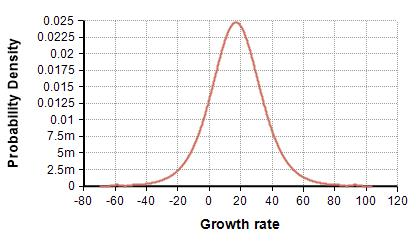# Logistic distribution

(Redirected from Logistic)

Release: 4.6  •  5.0  •  5.1  •  5.2  •  5.3  •  5.4  •  6.0  •  6.1  •  6.2  •  6.3  •  6.4

The logistic distribution describes a continuous, symmetric, smooth, uni-modal distribution with tails that are heavier than the normal distribution.

Logistic(17, 10)## Functions

### Logistic( mean, scale, over )

The distribution function. Use to define a quantity as being logistically-distributed.

### DensLogistic(x, mean, scale)

(New as a built-in function in Analytica 5.2)

The probability density at «x» for a logistic distribution with «mean» and «scale». Equal to

$\displaystyle{ p(x) = {\eta \over {s ( 1 + \eta)^2} } }$, where $\displaystyle{ \eta = \exp\left(-{ {x-mean}\over {scale}}\right) }$

### CumLogistic( x, mean, scale )

The cumulative density function, describing the probability of being less than or equal to «x». Given by

$\displaystyle{ F(x)=\frac{1}{1+exp \Big(-\frac{(x-mean)}{scale}\Big)} }$

### CumLogisticInv( p, mean, scale )

The inverse cumulative probability function, also know as the quantile function. Returns the value for which has a «p» probability of being greater than or equal to the true value.

$\displaystyle{ F^{-1}(p) = «mean» - «scale» \ln\left({1-p}\over p\right) }$

### Parameters

• «mean»: The mean, which for the logistic distribution is also the mode and median. Any real number.
• «scale»: optional, defaults to 1. Must be positive.
• «over»: optional. A list of indexes to independently sample over.

## Statistics

Theoretical (i.e., in the absence of sampling error) for the logistic distribution are as follows.

## Parameter Estimation

The parameters of the distribution can be estimated using:

«mean» := Mean(X, I)
«scale» := Sqrt(3*Variance(X, I))/Pi

## Applications

### LogisticRegression

The logistic distribution is particularly convenient for determining dependent probabilities using linear regression techniques, where the probability of a binomial event depends monotonically on a continuous variable x. For example, in a toxicology assay, x may be the dosage of a toxin, and p(x) the probability of death for an animal exposed to that dosage. Using p(x) = F(x), the logit of p, given by

Logit(p(x)) = Ln(p(x) / (1-p(x))) = x/s - m/s

has a simple linear form. This linear form lends itself to linear regression techniques for estimating the distribution — for example, from clinical trial data.

## Examples

Logistic(17, 10)